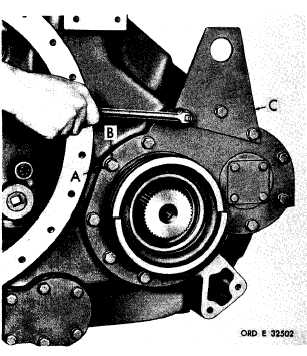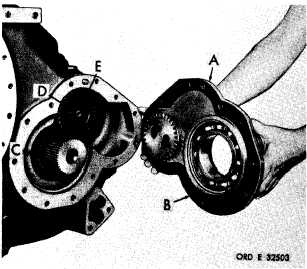Custom SearchP A R   5    S T E P S    1  9 - 2 2 D I S A S S E M B LY C H A P   5,   SEC   I I Figure   85   (Step   21) Remove   output   oil   pump   driven   gear   (A)   and k e y     ( B ) .     U s i n g     a     9 / 1 6 - i n c h     w r e n c h ,     r e m o ve four   self-locking   bolts   (C)   retaining   oil   pump ( D ) .    R e m o v e    o i l    p u m p    ( D ). Figure   83   (Step   19) Using   a   5/8-inch   wrench,   remove   13   bolts   (A), lock   washers   (B)   and   lifting   bracket   (C). Figure   84   (Step   20) Remove   left-output   support   assembly   (A),   gas- ket   (B)   and   output   oil   pump   drive   gear   (C). Remove   snap   ring   (D)   retaining   oil   pump   driven gear   (E). Figure   86   (Step   22) U s i n g    a    5 / 8 - i n c h    w r e n c h ,    r e m o v e    f i v e    c o n- v e r t e r - t o - m a i n     t r a n s m i s s i o n     h o u s i n g     a s s e m- b l y    b o l t s    ( A )    a n d    f l a t    w a s h e r s    ( B ) .    U s i n g    a 9 / 1 6 - i n c h    w r e n c h ,    r e m o v e    s i x    b o l t s    ( C )    a nd lock   washers   (D)   retaining   oil   screen   cover   (E). 8 9Integrated Publishing, Inc. - A (SDVOSB) Service Disabled Veteran Owned Small Business